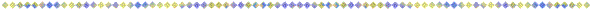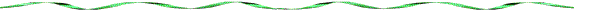宋明理學與陰陽學

1)

An^In→T4
i(kx-wt) -i(kx-wt)
T4(x,t)=Le
+Ge
2
│T4│=1

2
│T│=1

a)

b)

2)

a'vA'(a')
pvP(p)
[a'vA'(a')]^[pvP(p)]→
T1 稱為二氣交感，以心定義T1

i(kx-wt) -i(kx-wt)

3) 修養

1)

2)I^[InvAn]→
T4
i(kx-wt) -i(kx-wt)
T4(x,t)=Le      +Ge

i(kx-wt) -i(kx-wt)

i(kx-wt) -i(kx-wt)
T1(x,t)=Ae        +Pe

In^An→T4
i(kx-wt)    -i(kx-wt)
T4(x,t)=Le
+Ge

1.

2.

30.10.962012/7/24整理）